# Cars motion

From point A car start at the speed of 90 km/h. Against it departs from the point B at the same time another car at 60 km/h. For how long and where they met if the distance between A and B is 180 km?

t =  1.2 hod
x(A) =  108 km

### Step-by-step explanation:Did you find an error or inaccuracy? Feel free to write us. Thank you!Tips to related online calculators
Do you want to convert length units?
Do you want to convert velocity (speed) units?
Do you want to convert time units like minutes to seconds?

## Related math problems and questions:

• Motion 2At 10 o'clock started from place A car speed at 90 km/h. 20 minutes after started from the place B at speed 60 km/h another car. Distance places A and B is 120 kilometers. What time and where they meet?
• Cars 2Opava is 360 km distance from Prešov. At 8:00 hour, two cars started against each other from this cities at average speeds 88 km/h and 121 km/h. What hour will it meet and what distance it's travel if the car started from Prešov is faster?
• Car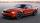The car goes from point A to point B at speed 86 km/h and back 53 km/h. If it goes there and back at speed 67 km/h trip would take 10 minutes shorter. What is the distance between points A and B?
• Cars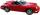Cars started at 7:00 AM. From point A car run at 40 km per hour, from B car run 70 km per hour. Between A and B is a 250 km long road. When and where will meet (measured from point A)?
• Brno-Tachov highwayThe distance Brno-Tachov is 360 km. At 8:00 a. M. , a car travels from Brno on the highway, traveling at an average speed of 90 km/h. An hour later, a car leaves Tachov towards Brno, whose average speed is 110 km/h. Calculate how far apart they will be at
• Two cars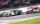Car A1 goes at average speed of 106 km/h and the second car A2 goes at 103 km/h. How many second will it take car A1 to circulate car A2? Assume that both cars are 5 meters long and the safety gap between cars is 1.5 meters.
• Two places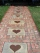The distance to the places is 60 km. From place A a pedestrian came out at a speed of 4 km/h and at the same time a car drove against him from place B. What was the speed of the car if the pedestrian met him in 90 minutes?
• Motorcyclist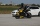From Trutnov, the motorcyclist started at an average speed of 60km/hour. At 12.30hrs the passenger car was started at a speed of 80km/hour. How many hours and at what distance from Trutnov will car catch a motorcycle?
• Against each other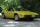From two points A, B distant 23 km at the same time started two cars against each other at speeds 41 km/h and 65 km/h. How long does cars meet and what distance passes each of them?
• CarThe driver of a car is to get to 608 km distant city. From atlas found that 162 km will have to pass through the cities at average speed 48 km/h. Remainder of the journey pass outside the cities at average speed 116 km/h. Calculate how many hours it will
• Cars speedsDistance from city A to city B is 108 km. Two cars were simultaneously started from both places. The speed of a car coming from city A was 2 km/h higher than the second car. What was the speed of each car if they met in 54 minutes?
• Two citiesCities A and B are 200 km away. At 7 o'clock from city A, the car started at an average speed of 80 km/h, and from B at 45 min later the motorcycle is started at an average speed of 120 km/h. How long will they meet and at what distance from the point A i
• MotionFrom two different locations distant 232 km started against car and bus. The car started at 5:20 with average speed 64 km/h. Bus started at 7:30 with average speed 80 km/h. When they meet? How many kilometers went the bus?
• Average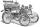What is the average speed of the car, where half of the distance covered passed at speed 66 km/h and the other half at 86 km/h.
• Motion problemFrom Levíc to Košíc go car at speed 81 km/h. From Košíc to Levíc go another car at speed 69 km/h. How many minutes before the meeting will be cars 27 km away?
• Train and car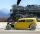The train and the car started at a constant speed to journey. When the train travels 87 km, the car travels 97 km. How many km travels the train when the car travels 87 km?
• Where and when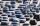The truck left Kremnica at 11:00 h at a speed of 60km/h. At 12:30 h, the passenger car started at an average speed of 80km/h. How many kilometers from Kremnica will the passenger car reach the truck, and when?